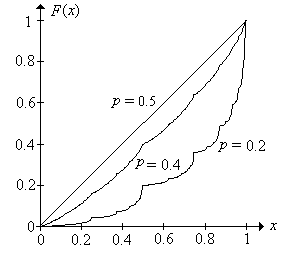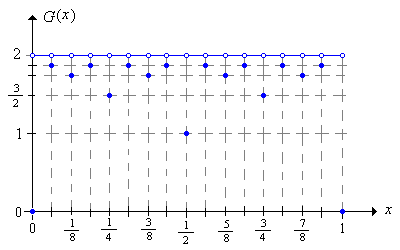]> Bold Play

10. Bold Play

Recall that with the strategy of bold play in red and black, the gambler on each game bets either her entire fortune or the amount needed to reach the target fortune, whichever is smaller. As usual, we are interested in the probability that the player reaches the target and the expected number of trials. The first interesting fact is that only the ratio of the initial fortune to the target fortune matters, quite in contrast to timid play.

Suppose that the gambler plays boldly with initial fortune $x$ and target fortune $a$. As usual, let $X X 0 X 1$ denote the fortune process for the gambler. Argue that for any $c 0$, the random process $c X c X 0 c X 1$ is the fortune process for bold play with initial fortune $c x$ and target fortune $c a$.

Because of this result, it is convenient to use the target fortune as the monetary unit and to allow irrational, as well as rational, initial fortunes. Thus, the fortune space is $0 1$. Sometimes in our analysis we will ignore the states 0 or 1; clearly there is no harm in this because in these states, the game is over.

Recall that the betting function $S$ is the function that gives the amount bet as a function of the current fortune. Show that

$S x x 1 x x 0 x 12 1 x 12 x 1$The Probability of Winning

We will denote the probability that the bold gambler reaches the target $a 1$ starting from the initial fortune $x 0 1$ by $F x$. By Exercise 1, the probability that the bold gambler reaches some other target value $a$, starting from $x 0 a$ is $F x a$.

By conditioning on the outcome of the first game, show that $F$ satisfies the functional equation in part (a) and boundary conditions in part (b):

1. $F x p F 2 x x 0 12 p q F 2 x 1 x 12 1$
2. $F 0 0 , F 1 1$

From the prvious exericse, and a little thought, it should be clear that an important role is played by the function $d$ defined on $0 1$ by

$d x 2 x 2 x 2 x x 0 12 2 x 1 x 12 1$

The function $d$ is sometimes called the doubling function, mod 1, since $d x$ gives the fractional part of $2 x$. Note that until the last bet that ends the game (with the player ruined or victorious), the successive fortunes of the player follow iterates of the map $d$. Thus, bold play is intimately connected with the dynamical system associated with $d$.Binary Expansions

One of the keys to our analysis is to represent the initial fortune in binary form. The binary expansion of $x 0 1$ is

$x i 1 x i 2 i$

where $x i 0 1$ for each $i$. This representation is unique except when $x$ is a binary rational (sometimes also called a dyadic rational), that is, a number of the form $k 2 n$ where $n$ and $k 1 3 2 n 1$; the positive integer $n$ is called the rank of $x$. For a binary rational $x$ of rank $n$, we will use the standard terminating representation where $x n 1$ and $x i 0$ for $i n$. Rank can be extended to all numbers in [0, 1) by defining the rank of 0 to be 0 (0 is also considered a binary rational) and by defining the rank of a binary irrational to be . We will denote the rank of $x$ by $r x$.

Applied to the binary sequences, the doubling function $d$ is the shift operator:

Show that $d x i x i 1$ for $x 0 1$

Bold play in red and black can be elegantly described by comparing the bits of the inital fortune with the game bits.

Suppose that gambler starts with initial fortune $x 0 1$. Show that the gambler eventually reaches the target 1 if and only if there exists a positive integer $k$ such that $I j 1 x j$ for $j 1 2 k 1$ and $I k x k$. That is, the gambler wins if and only if when the game bit agrees with the corresponding fortune bit for the first time, that bit is 1.

The random variable whose bits are the complements of the fortune bits will play an important role in our analysis. Thus, let

$W i 1 1 I j 2 j$

Note that $W$ is a well defined random variable taking values in $0 1$.

Suppose that the gambler starts with initial fortune $x 0 1$. Use the result of the previous exercise to show that the gambler reaches the target 1 if and only if $W x$.

Show that $W$ has a continuous distribution. That is, show that $W x 0$ for any $x 0 1$.

From the previous two exercises, it follows that $F$ is simply the distribution function of $W$. In particular, $F$ is an increasing function, and since $W$ has a continuous distribution, $F$ is a continuous function.

Show that the success function $F$ is the unique continuous solution of the functional equation in Exercise 3.

1. Use induction on the rank to show that any two solutions must agree at the binary rationals.
2. Use part (a) and continuity to show that any two continuous solutions must agrre for all $x$.

If we introduce a bit more notation, we can give nice expression for $F x$, and later for the expected number of games $G x$. Let $p 0 p$ and $p 1 q 1 p$.

Show that

$F x n 1 p x 1 p x n 1 p x n$

Show that $F$ is strictly increasing on $0 1$. This means that the distribution of $W$ has support $0 1$; that is, there are no subintervals of $0 1$ that have positive length, but 0 probability.

In particular, show that

1. $F 18 p 3$
2. $F 28 p 2$
3. $F 38 p 2 p 2 q$
4. $F 48 p$
5. $F 58 p p 2 q$
6. $F 68 p p q$
7. $F 78 p p q p q 2$

Suppose that $p 12$. Show that $F x x$ for $x 0 1$ in two ways:

1. Using the functional equation in Exericse 3.
2. Using the representation in Exercise 10.

Thus, for $p 12$ (fair trials), the probability that the bold gambler reaches the target fortune $a$ starting from the initial fortune $x$ is $x a$, just as it is for the timid gambler. Note also that the random variable $W$ has the uniform distribution on $0 1$. When $p 12$, the distribution of $W$ is quite strange. To state the result succinctly, we will indicate the dependence of the of the probability measure  on the parameter $p 0 1$. First we define

$C p x 0 1 1 n i 1 n 1 x i p as n$

Thus, $C p$ is the set of $x 0 1$ for which the relative frequency of 0's in the binary expansion is $p$.

Use the strong law of large numbers to show that

1. $p W C p 1$ for $p 0 1$
2. $p W C t 0$ for $p t$

Show that when $p 12$, $W$ does not have a probability density function, even though it has a continuous distribution. The following steps outline a proof by contradiction

1. Suppose that $W$ has probability density function $f$
2. Then $p W C p x C p f x$.
3. By the previous exercise, $p W C p 1$.
4. But also $x C p 1 12 W C p 0$. That is, $C p$ has Lebesgue measure 0.
5. Hence $x C p f x 0$.

When $p 12$, $F$ has derivative 0 at almost every point in $0 1$, even though it is strictly increasing. The following picture shows the graphs of $F$ when $p 0.5$, $p 0.4$, and $p 0.2$.In the red and black experiment, select Bold Play. Vary the initial fortune, target fortune, and game win probability with the scroll bars and note how the probability of winning the game changes. In particular, note that this probability depends only on $x a$. Now for various values of the parameters, run the experiment 1000 times with an update frequency of 100 and note the apparent convergence of the relative frequency function to the probability density function.

The Expected Number of Trials

Let $G x N X 0 x$ for $x 0 1$, the expected number of trials starting at $x$. For any other target fortune $a 0$, the expected number of trials starting at $x 0 a$ is just $G x a$.

By conditioning on the outcome of the first game, show that $G$ satisfies the following functional equation in (a) and boundary conditions (b):

1. $G x 1 p G 2 x x 0 12 1 q G 2 x 1 x 12 1$
2. $G 0 0 , G 1 0$

Note, interestingly, that the functional equation is not satisfied at $x 0$ or $x 1$. As before, we can give an alternate analysis using the binary representation of an initial fortune $x 0 1$.

Suppose that the initial fortune of the gambler is $x 0 1$. Show that $N k I k x k k r x$.

1. If $x$ is a binary rational then $N$ takes values in the set $1 2 r x$. Play continues until the game number agrees with the rank of the fortune or a game bit agrees with the corresponding fortune bit, whichever is smaller. In the first case, the penultimate fortune is $12$, the only fortune for which the next game is always final.
2. If $x$ is a binary irrational then $N$ takes values in . Play continues until a game bit agrees with a corresponding fortune bit.

We can give an explicit formula for the expected number of trials $G x$ in terms of the binary representation of $x$.

Suppose that $x 0 1$, and recall our special notation: $p 0 p$, $p 1 q$. Show that

$G x n 0 r x 1 p x 1 p x n$
1. Note that the $n 0$ term is 1, since the product is empty.
2. The sum has a finite number of terms if $x$ is a binary rational.
3. The sum has an infinte number of terms if $x$ is a binary irrational.

Use the result of the last exercise to verify the following values:

1. $G 18 1 p p 2$
2. $G 28 1 p$
3. $G 38 1 p p q$
4. $G 18 1$
5. $G 58 1 q p q$
6. $G 68 1 q$
7. $G 78 1 q q 2$

Suppose that $p 12$. Show that

$G x 2 1 2 r x 1 x is binary rational 2 x is binary irrational$In the red and black experiment, select Bold Play. Vary $x$, $a$, and $p$ with the scroll bars and note how the expected number of trials changes. In particular, note that the mean depends only on the ratio $x a$. For selected values of the parameters, run the experiment 1000 times with an update frequency of 100 and note the apparent convergence of the sample mean to the distribution mean.

For fixed $x$, show that $G$ is continuous as a function of $p$.

However, as a function of the initial fortune $x$, for fixed $p$, the function $G$ is very irregular.

Show that $G$ is discontinuous at the binary rationals in $0 1$ and continuous at the binary irrationals.